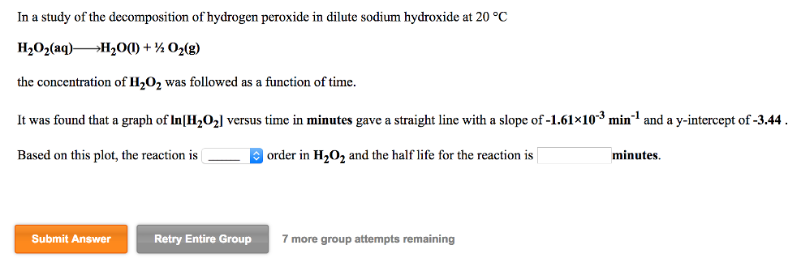# Problem: In a study of the decomposition of hydrogen peroxide in dilute sodium hydroxide at 20°C. H2O2 (aq) →H2O (l) + 1/2O2 (g) the concentration of H2O2 was followed as a function of time. It was found that a graph of ln[H2O2] versus time in minutes gave a straight line with a slope of -1.61 x 10-3 min-1 and a y-intercept of -3.44. Based on this plot, the reaction is ______________ order in H2O2 and the half life for the reaction is ____________ minutes.

###### FREE Expert Solution
92% (202 ratings)###### Problem Details

In a study of the decomposition of hydrogen peroxide in dilute sodium hydroxide at 20°C.

H2O(aq) →H2O (l) + 1/2O(g)

the concentration of H2O2 was followed as a function of time.

It was found that a graph of ln[H2O2] versus time in minutes gave a straight line with a slope of -1.61 x 10-3 min-1 and a y-intercept of -3.44.

Based on this plot, the reaction is ______________ order in H2O2 and the half life for the reaction is ____________ minutes.